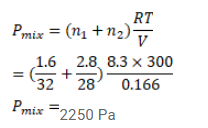# Use R=8.3 J/mol-K wherever required. A vessel contains 1.60 g of oxygen andQuestion:

Use $\mathrm{R}=8.3 \mathrm{~J} / \mathrm{mol}-\mathrm{K}$ wherever required.

A vessel contains $1.60 \mathrm{~g}$ of oxygen and $2.80 \mathrm{~g}$ of nitrogen. The temperature is maintained at $300 \mathrm{~K}$ and volume of the vessel is $0.166 \mathrm{~m}^{3}$. Find the pressure of the mixture.

Solution: